Next: Connection to coding Up: Ensemble learning Previous: Ensemble learning

## Model selection in ensemble learning

Recall that the cost function Cy(x | H) can be translated into lower bound for p(x | H). Since p(H | x) = p(x | H) p(H) / p(x), it is natural that Cy(x | H) can be used for model selection also by equating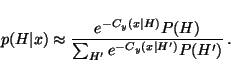(7)

In fact, we can show that the above equation gives the best approximation for p(H | x) in terms of Cy, H(x), the Kullback-Leibler divergence between q(y, H | x) and p(y, H | x), which means that the model selection can be done using the same principle of approximating the posterior distribution as learning parameters.

Without losing any generality from q(y, H | x), we can write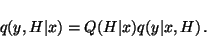(8)

Now the cost function can be written as

 Cy, H(x) =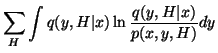=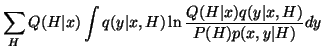=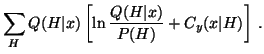(9)

Minimising Cy, H(x) with respect to Q(H | x) under the constraint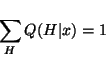(10)

yields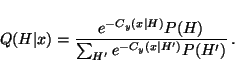(11)

Substituting this into equation 9 yields the minimum value for Cy, H(x) which is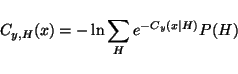(12)

If we wish to use only a part of different model structures H, we can try to find those H which would minimise Cy, H(x). It is easy to see that this is accomplished by choosing the models corresponding to Cy(x | H). A special case is to use only one Hcorresponding to the smallest Cy(x | H).Next: Connection to coding Up: Ensemble learning Previous: Ensemble learning
Harri Lappalainen
2000-03-03